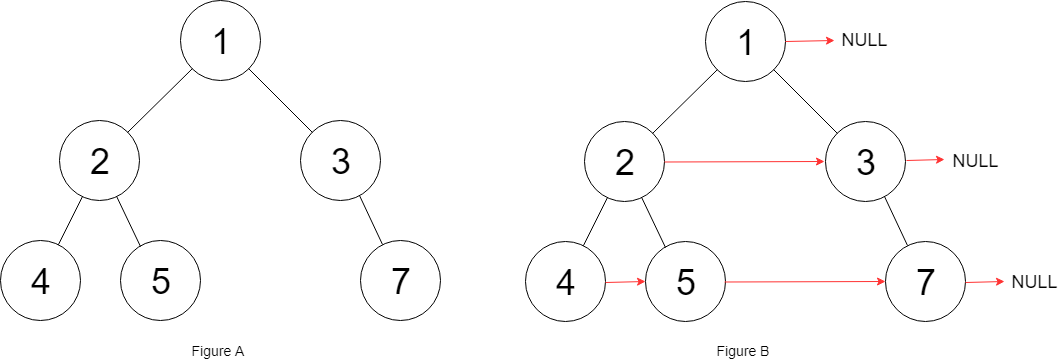엔지니어 게시판
LeetCode 솔루션 분류

# [5/13] 117. Populating Next Right Pointers in Each Node II

• mingki 작성
• 작성일

• 280 조회
• 5 댓글

### 본문

[LeetCode 시즌 3] 2022년 5월 13일 문제입니다.

117. Populating Next Right Pointers in Each Node II
Medium

Given a binary tree

```struct Node {
int val;
Node *left;
Node *right;
Node *next;
}
```

Populate each next pointer to point to its next right node. If there is no next right node, the next pointer should be set to `NULL`.

Initially, all next pointers are set to `NULL`.

Example 1:```Input: root = [1,2,3,4,5,null,7]
Output: [1,#,2,3,#,4,5,7,#]
Explanation: Given the above binary tree (Figure A), your function should populate each next pointer to point to its next right node, just like in Figure B. The serialized output is in level order as connected by the next pointers, with '#' signifying the end of each level.
```

Example 2:

```Input: root = []
Output: []
```

Constraints:

• The number of nodes in the tree is in the range `[0, 6000]`.
• `-100 <= Node.val <= 100`

Follow-up:

• You may only use constant extra space.
• The recursive approach is fine. You may assume implicit stack space does not count as extra space for this problem.

댓글 5

## mingki님의 댓글

• mingki
• 작성일
C++
Runtime: 15 ms, faster than 73.10% of C++ online submissions for Populating Next Right Pointers in Each Node II.
Memory Usage: 17.6 MB, less than 44.54% of C++ online submissions for Populating Next Right Pointers in Each Node II.
``````class Solution {
public:
Node* connect(Node* root) {
queue<Node*> q;

if (!root) return root;
q.push(root);
while (!q.empty()) {
int size = q.size();
for (int i = 0; i < size; ++i) {
Node *tmp = q.front(); q.pop();
tmp->next = i == size - 1 ? NULL : q.front();
if (tmp->left) q.push(tmp->left);
if (tmp->right) q.push(tmp->right);
}
}
return root;
}
};``````

## austin님의 댓글

• austin
• 작성일
``````class Solution {
public:
Node* connect(Node* root) {
for(vector<Node*> cur{root}, tmp; root && !cur.empty(); cur = move(tmp)) {
Node* prev = nullptr;
for(auto n : cur) {
if (prev) prev->next = n;
if (n->left) tmp.emplace_back(n->left);
if (n->right) tmp.emplace_back(n->right);
prev = n;
}
}
return root;
}
};``````

## bohuim님의 댓글

• bohuim
• 작성일
``````class Solution {
var map = [Node?]()

func connect(_ root: Node?) -> Node? {
guard let node = root else { return nil }
return connect(node, depth: 0)
}

func connect(_ node: Node, depth: Int) -> Node {
// If we haven't seen this depth yet, append a nil.
let nextDepth = depth + 1
if map.count < nextDepth {
map.append(nil)
}

// Do a reverse order traversal.
if let right = node.right {
connect(right, depth: nextDepth)
}

node.next = map[depth]
map[depth] = node

if let left = node.left {
connect(left, depth: nextDepth)
}
return node
}
}``````

## Coffee님의 댓글

• Coffee
• 작성일
``````/*
// Definition for a Node.
class Node {
public int val;
public Node left;
public Node right;
public Node next;

public Node() {}

public Node(int _val) {
val = _val;
}

public Node(int _val, Node _left, Node _right, Node _next) {
val = _val;
left = _left;
right = _right;
next = _next;
}
};
*/

class Solution {
public Node connect(Node root) {

if(root == null){return root;}

while(queue.size() > 0){
int size = queue.size();        // size is level
for(int i=0; i<size; i++){
Node node = queue.poll(); // check

if(i < size - 1){       // connect the nodes at current levels.
node.next = queue.peek();
}

if(node.left != null){
queue.offer(node.left);
}

if(node.right != null){
queue.offer(node.right);
}
}
}

return root;

}
}``````

## 나무토끼님의 댓글

• 나무토끼
• 작성일
Runtime: 82 ms, faster than 26.79% of Python3 online submissions for Populating Next Right Pointers in Each Node II.
Memory Usage: 15.3 MB, less than 48.78% of Python3 online submissions for Populating Next Right Pointers in Each Node II.

``````class Solution:
def connect(self, root: 'Node') -> 'Node':
if not root:
return root
que = []
que.append(root)
while que:
size = len(que)
for i in range(1, size):
que[i - 1].next = que[i]
for i in range(size):
front = que.pop(0)
if front.left:
que.append(front.left)
if front.right:
que.append(front.right)
return root``````
전체 85 / 1 페이지

• 등록일 05.21
• 등록일 05.21
• 등록일 05.21
• 등록일 05.21
• 등록일 05.21
• 등록일 05.21
• 등록일 05.21
• 등록일 05.21
• 등록일 05.21

### 새댓글

• 등록자 뚝배기 등록일 05.21
• 등록자 coderoncruise 등록일 05.21
• 등록자 coderoncruise 등록일 05.21
• 등록자 Connie 등록일 05.21
• 등록자 JakeMinSVK 등록일 05.21
• 등록자 JakeMinSVK 등록일 05.21
• 등록자 Connie 등록일 05.20
• 등록자 Jack 등록일 05.20
• 등록자 Connie 등록일 05.19
• 등록자 Connie 등록일 05.19

### Stats

• 현재 접속자 82 명
• 오늘 방문자 141 명
• 어제 방문자 938 명
• 최대 방문자 1,221 명
• 전체 회원수 216 명